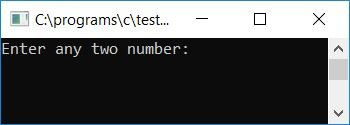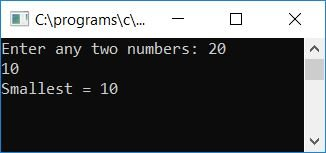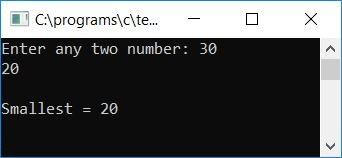# C program to find the smallest of two numbers

Here we will learn about how to find and print the smallest number between any two given numbers (by the user at run-time) with and without using a user-defined function. At last, we will also use the ternary operator to find and print the smallest between the two numbers.

## Find the smaller of two numbers in C without a user-defined function

Here is the program that will ask the user to enter any two numbers to find out and print the smallest one, as shown here:

```#include<stdio.h>
#include<conio.h>
int main()
{
int a, b, small;
printf("Enter any two number: ");
scanf("%d%d", &a, &b);
if(a<b)
small=a;
else
small=b;
printf("\nSmallest of the two number is: %d", small);
getch();
return 0;
}```

The program was written in the Code::Blocks IDE; therefore, after a successful build and run, here is the first snapshot of the sample run of the above program:Supply any two numbers and press the ENTER key to see the smallest number between the given two numbers, as shown here in the second snapshot:Here are some of the main steps used in the above program:

• Receive any two numbers as input.
• Use an if statement to check whether the first number is less than the second number or not.
• If it is, then initialize the first number to any variable, say small.
• If it does not, then go to the else block and initialize the value of the second number to any variable, say small.
• Print the value of small; that will be the smallest number between the given two.

## C function to return the smaller of two numbers

Here is another program using functions. In this program, we've written a function that takes two numbers as arguments and returns the smaller of the two.

```#include<stdio.h>
#include<conio.h>
int findSmall(int, int);
int main()
{
int num1, num2, small;
printf("Enter any two numbers: ");
scanf("%d%d", &num1, &num2);
small = findSmall(num1, num2);
printf("Smallest = %d", small);
getch();
return 0;
}
int findSmall(int a, int b)
{
if(a>b)
return b;
else
return a;
}```

Here is the final snapshot of the sample run:## C Using the Ternary Operator, find the smaller of two numbers

Now let's create another program that will also do the same task as the above two programs, that is, find out and print the smallest of any two numbers, but this time I'll use the ternary operator in this case.

This is the shortest program to find the smallest number between any two numbers, as we have used the ternary operator here.

```#include<stdio.h>
#include<conio.h>
int main()
{
int a, b, small;
printf("Enter any two number: ");
scanf("%d%d", &a, &b);
small = (a<b) ? a : b;
printf("\nSmallest = %d", small);
getch();
return 0;
}```

Here is the final snapshot of the sample run of this program:The main logic of the above program is:

`small = (a<b) ? a : b;`

Here, if the expression a<b (if a is less than b) is true, then the value of a will be initialized to small. Otherwise, the value of b will be set to small. That is, if a is less than b, then the expression will become:

`small = a;`

Otherwise, if a is not less than b or b is less than a, then the expression will become:

`small = b;`

C Quiz

« Previous Program Next Program »